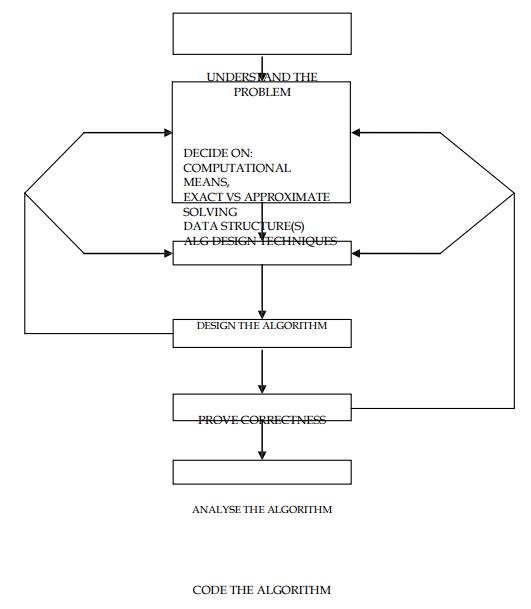Home | | User Interface Design | Introduction and Definition of Algorithm

# Introduction and Definition of Algorithm

Algorithmic is more than the branch of computer science. It is the core of computer science, and, in all fairness, can be said to be relevant it most of science, business and technology

ALGORITHM ANALYSIS

Introduction

An algorithm is a sequence of unambiguous instruction for solving a problem, for obtaining a required output for any legitimate input in a finite amount of time.

Definition

â€śAlgorithmic is more than the branch of computer science. It is the core of computer science, and, in all fairness, can be said to be relevant it most of science, business and technologyâ€ť

Understanding of Algorithm

An algorithm is a sequence of unambiguous instruction for solving a problem, for obtaining a required output for any legitimate input in a finite amount of time.ALGORITHM DESIGN AND ANALYSIS PROCESSStudy Material, Lecturing Notes, Assignment, Reference, Wiki description explanation, brief detail
Design and Analysis of Algorithm : Introduction and Definition of Algorithm |